# Fraction Calculator

Created by Hanna Pamuła, PhD candidate
Reviewed by Dominik Czernia, PhD candidate and Jack Bowater
Last updated: Apr 06, 2022

Welcome to our fraction calculator, a really versatile tool. It can:

subtract,

✖️multiply, and

divide
any two fractions. Moreover, it has the ability to simplify a fraction (A.K.A. reduce), as well as tell you how to turn a fraction into decimal, and vice versa. If you're still not impressed, this tool not only works on standard proper and improper fractions, but also on mixed fractions - what more could you ask for?😁

As usual, we've prepared some introductory reading, which will let you dig into the world of fractions🌎. Below you'll read about the fraction definition, the types of fractions (proper, improper, mixed), and all those basic operations on simple and mixed fractions. Are you ready?

## What is a fraction? Fraction definition

A fraction represents the number of equal parts of a whole thing.
You can recognize a simple fraction because it has two numbers separated by line (or slash):

• We call the top number the numerator, and write it above the line. It tells us

How many parts we have

• We call the bottom number denominator, and display it below the line. It means

The total number of parts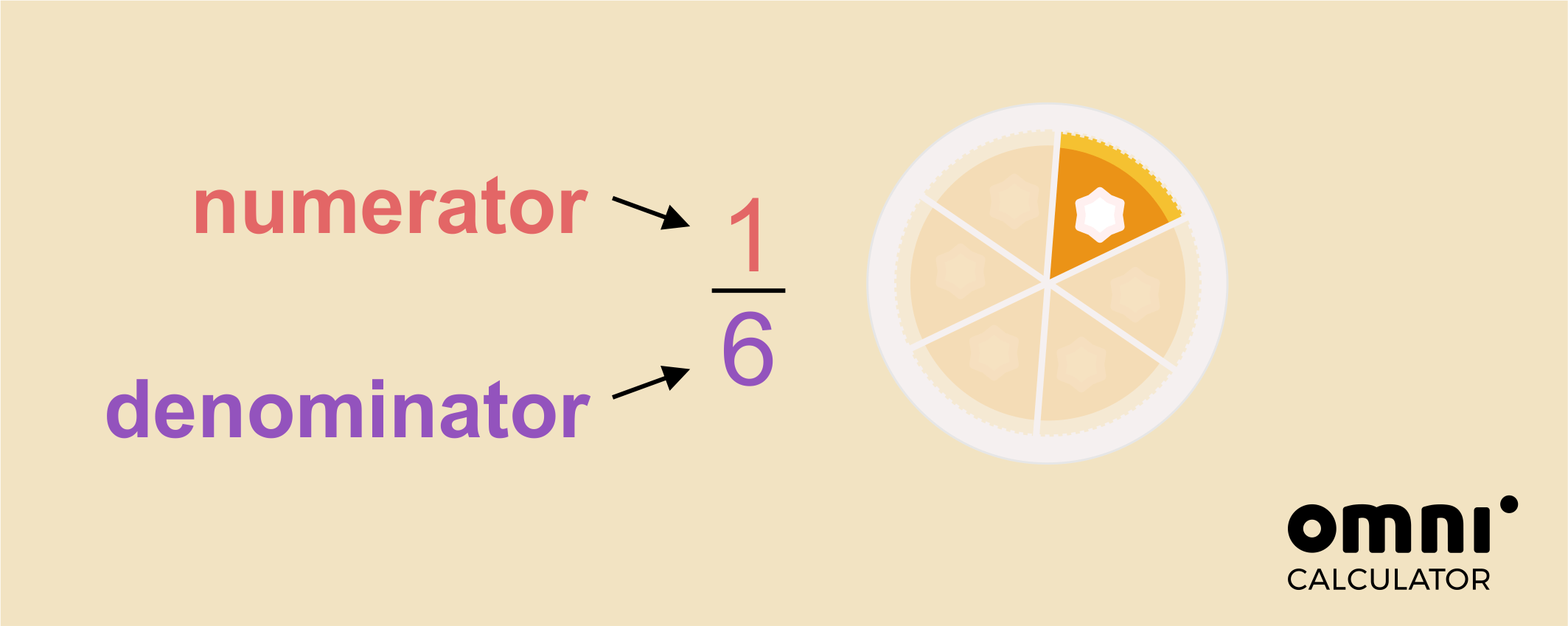In the example above, it's one part out of six slices, which the pie was cut into in total. We read it as one-sixth of the whole pie.

But, of course, the cake can be cut differently!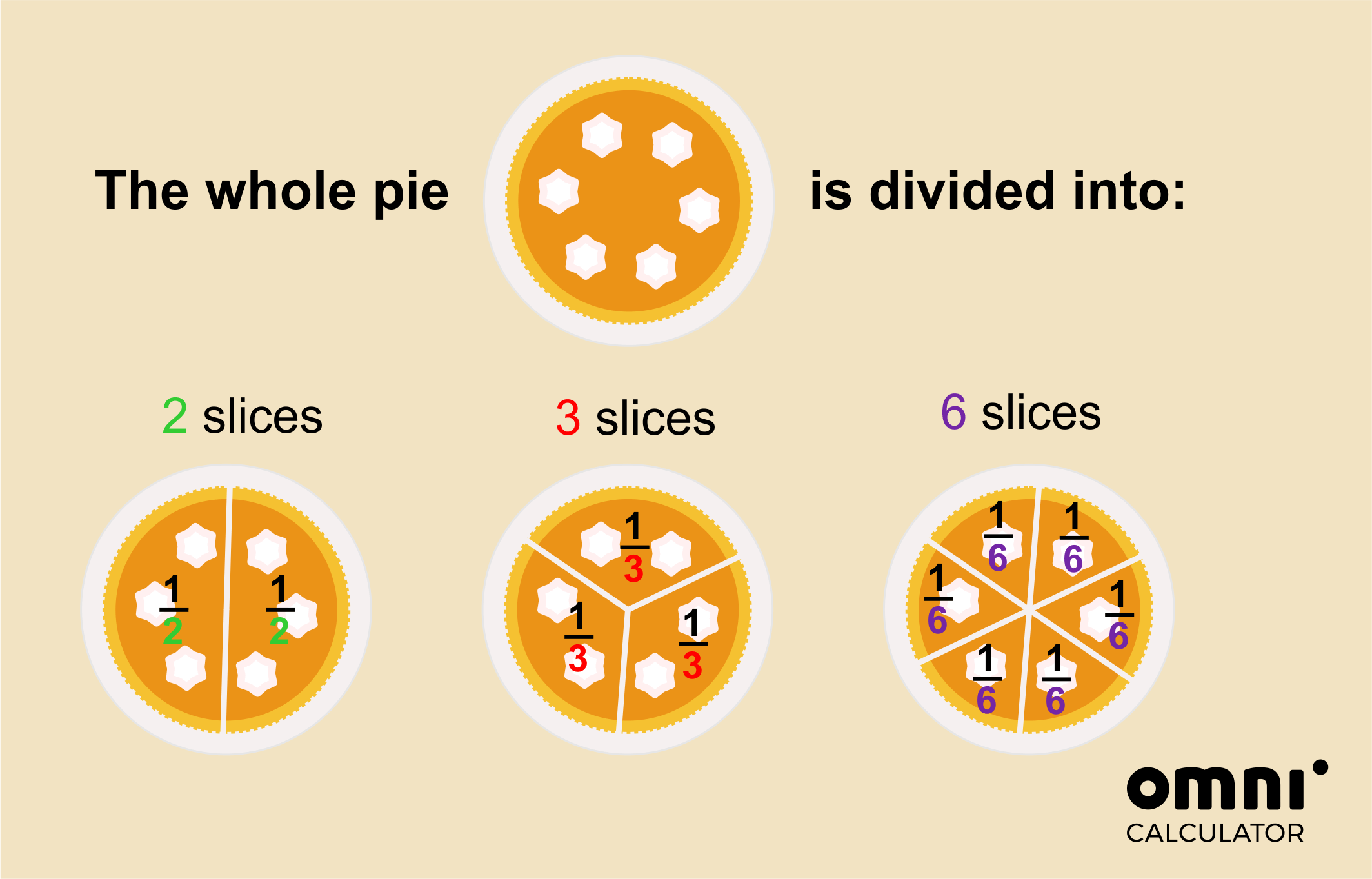So, if you cut the pie in 2, then one slice is one-half of the whole. If you cut the pie in 3 parts, then one slice is one-third of the whole pie, etc.

Fractions are used all around us:

## What is a proper, improper, and mixed fraction?

So, now that you know the fraction definition, let's have a look at the different types of fractions. In general, we have three types of fractions: proper, improper and mixed fractions:

1. Proper fraction

In proper fractions, the top number (numerator) is smaller than the bottom number (denominator). It means that it will always be less than one whole thing, e.g.:

🍰 5 slices of cake out of a cake that was cut into 6;

🍫 2 rows of a chocolate bar out of the whole chocolate bar, which has 5 rows; and

🍊 7 parts of an orange out of the entire orange, that we cut into 8.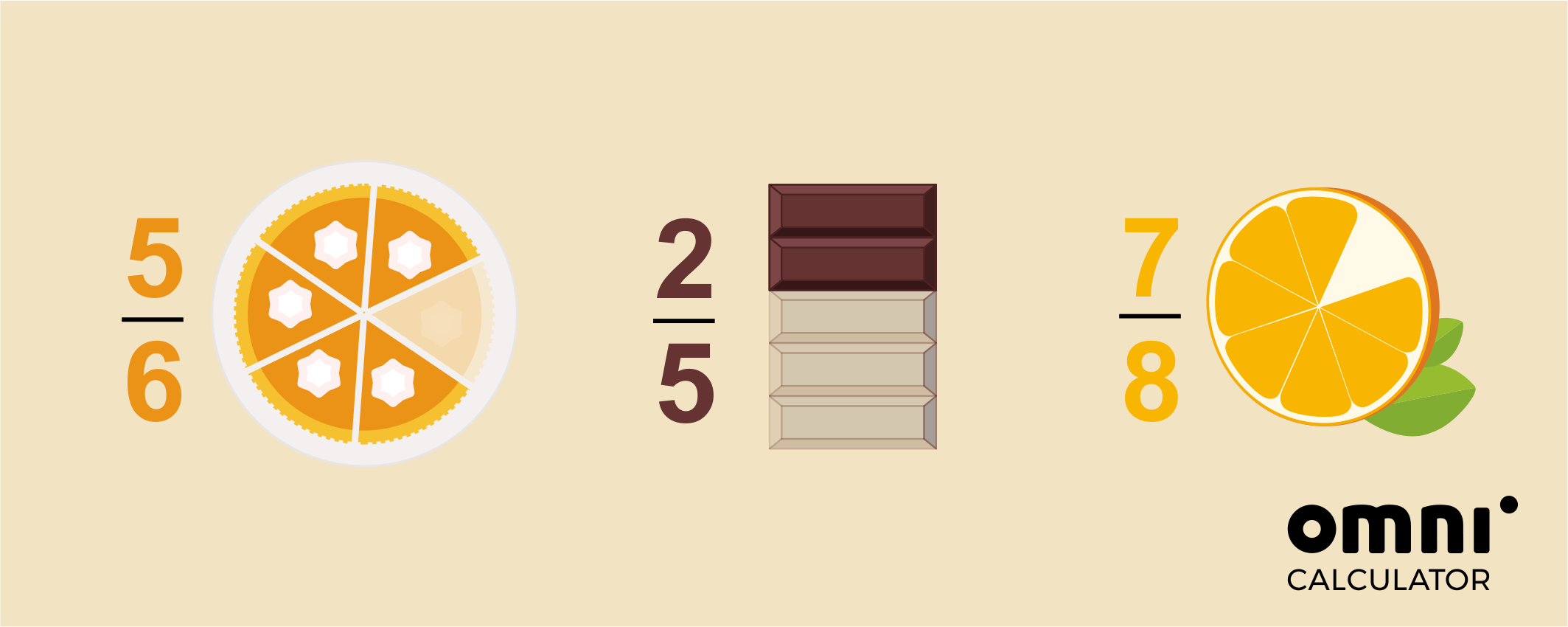The general rule, which works for both positive and negative numbers, is that the absolute value of the fraction is less than one:

|numerator/denominator|< 1

1. Improper fraction

So what is an improper fraction? It's a fraction where the numerator is larger than (or equal to) the denominator. Improper fractions are sometimes called top-heavy fractions. Examples of improper fractions are:

🍰 10 slices of cake, when each cake has 6 slices;

🍫 8 rows of a chocolate bar. A whole chocolate bar has 5 rows; and

🍊 21 parts of orange, if we cut each orange to 8 equal pieces.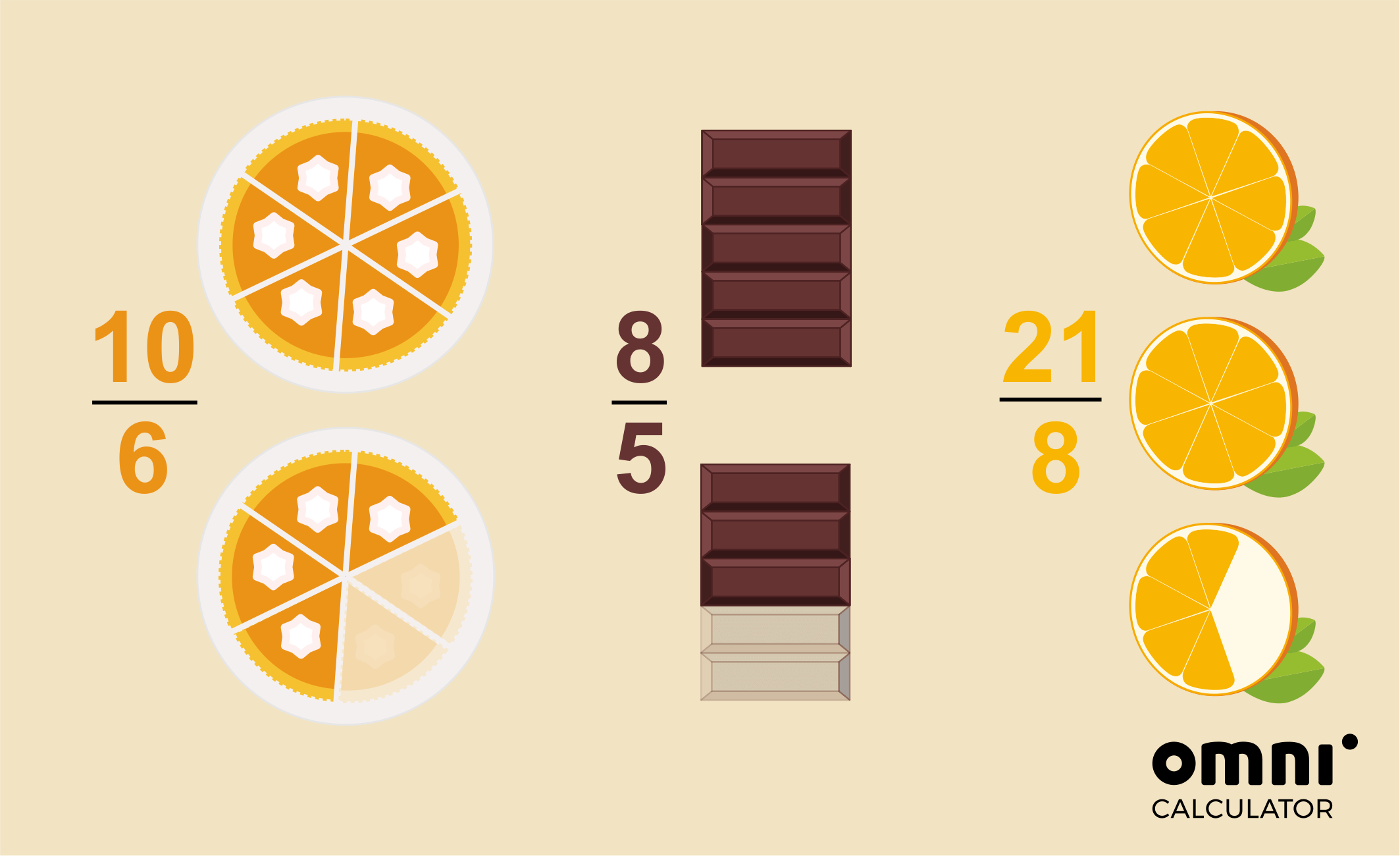1. Mixed fraction

Mixed fractions, also known as mixed numerals or mixed numbers, are another way of expressing an improper fraction.
They are whole numbers (the amount of whole things) and a proper fraction put together. So, if we'll take a look at the examples from the previous paragraph:

🍰 10 slices of cake, when each cake has 6 slices is the same as → 1 whole cake and 4 slices out of 6;

🍫 8 rows of chocolate when the whole chocolate bar has 5 rows1 whole chocolate bar and 3 rows out of 5; and

🍊 21 parts of orange, if we cut the orange to 8 slices2 whole oranges and five slices out of 8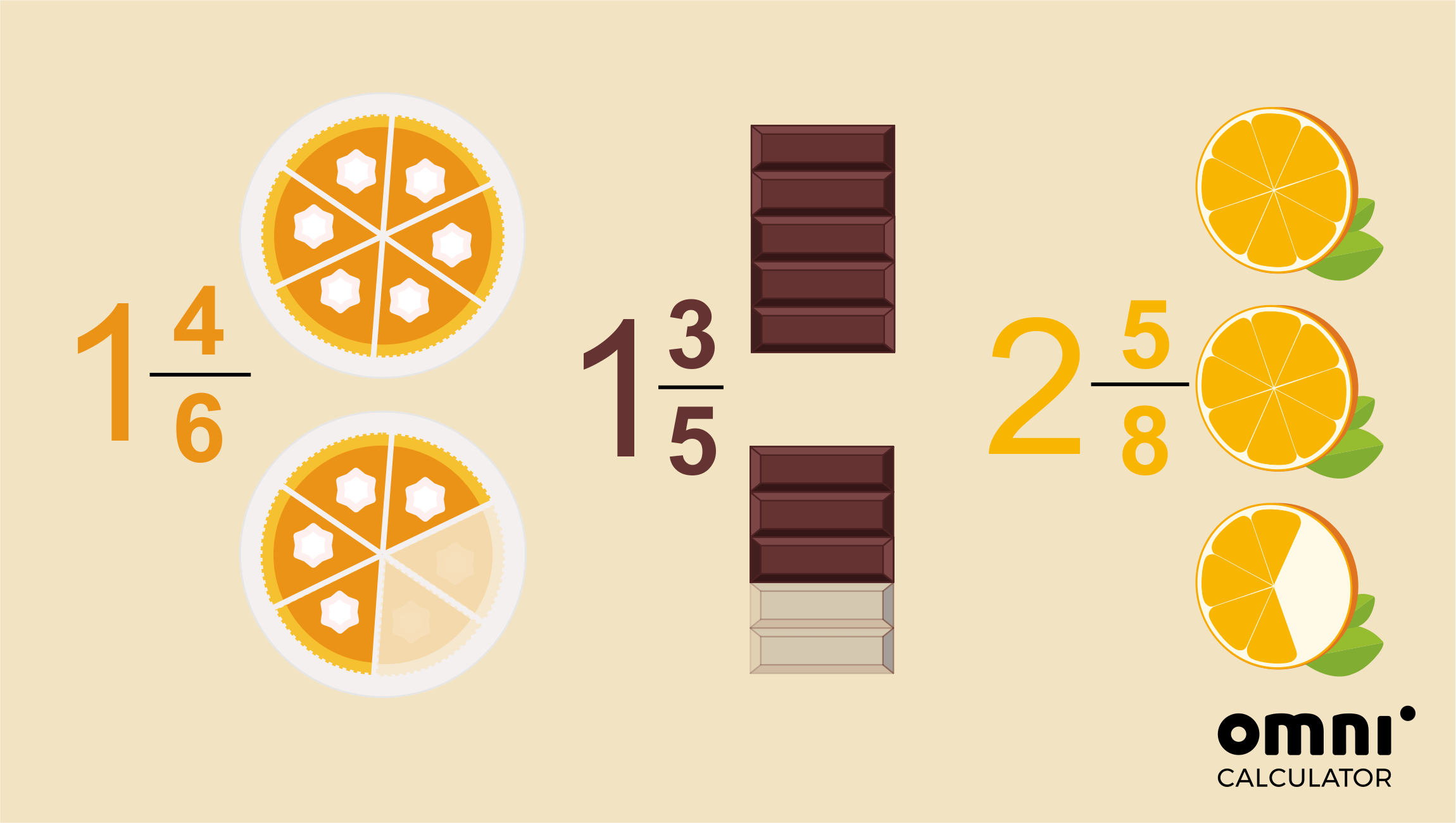💡 The most important things to remember after reading this section: • A proper fraction has a smaller top number (numerator) than its bottom number (denominator); • An improper fraction's numerator is larger than (or equal to) its denominator; and • A mixed number consists of a whole number and a proper fraction.

When it comes to adding fractions, there are three scenarios:

1. The denominator (bottom number) is the same in both fractions - e.g., 3/5 and 1/5

This is the most straightforward case; all you need to do is to add numerators (top numbers) together and leave the denominator as is, e.g:

3/5 + 1/5 = (3 + 1)/5 = 4/5

1. The fractions have unlike denominators - e.g., 2/5 and 3/10

This is a bit more of a complicated case - to add these fractions, you need to find the common denominator.

LCM(5,10) = 10

The other option is to multiply your denominators and reduce the fraction later.

• Then, you need to expand each fraction, to have this common denominator as its bottom number:

So, you should multiply the fraction with the denominator equal to 5 (our 1/5) by 2 to get 10 (remember that you must multiply both top and bottom numbers):

2/5 = (2 * 2)/(5 * 2) = 4/10

3/10

• Now that your fractions have the same denominator, you can add them:

4/10 + 3/10 = (4 + 3)/10 = 7/10

1. You want to add two mixed fractions - e.g. 2 3/5 and 1 1/2

One solution for this kind of problem is to convert the mixed fraction to an improper fraction and sum it as usual.

• Let's convert it for 2 3/5
• Multiply the whole number by the denominator:

2 * 5 = 10

• Add the result to you numerator:

10 + 3 = 13

• That's your new numerator - write it on top of your denominator:

2 3/5 = 13/5

Analogically, you can find out that 1 1/2 = 3/2

• Do the standard addition of fractions with uneven denominators:

13/5 + 3/2 = 26/10 + 15/10 = 41/10

• Finally, you can convert your result back into a mixed fraction:

Do long division with a remainder:

41/10 = 4 R 1

So 41/10 = 4 1/10

Of course, our fraction calculator deals with all of these scenarios. 😎

If you're still wondering how adding fractions works, maybe this visual will help?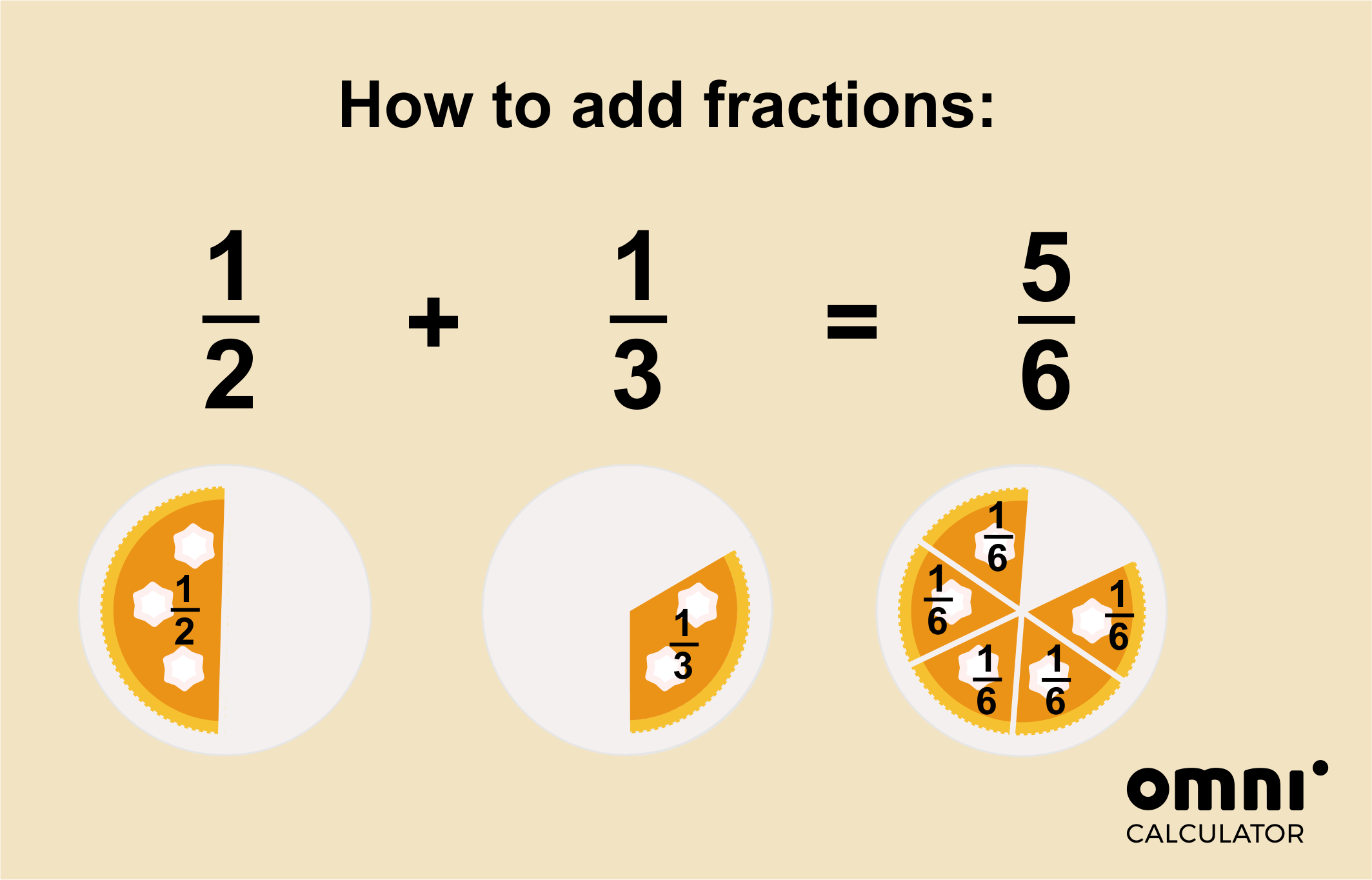For an even more thorough explanation, check out our adding fractions calculator.

## How to subtract fractions ➖

If you're wondering how to subtract fractions, and you've read through the previous section , we have some good news for you: it's pretty much the same!

1. If you have fractions with the same denominator, subtract the numerators:

3/5 - 1/5 = (3 - 1)/5 = 2/5

1. When subtracting fractions with unlike denominators - 2/5 and 3/10 - repeat the procedure from the previous section, but subtracting, not adding in the final step:
• Find a common denominator - it's 10.
• Expand the fractions to their equivalent fractions with a common denominator: 4/10 and 3/10.
• Subtract the numerators.

2/5 - 3/10 = 4/10 - 3/10 = 1/10

1. For mixed fractions (2 3/5 and 1 1/2):
• Change the mixed fractions to improper fractions, as before

2 3/5 = 13/5 and 1 1/2 = 3/2

• Subtract the two improper fractions with unequal denominators:

13/5 - 3/2 = 26/10 - 15/10 = 11/10

• Leave it in an improper fraction, or convert it back to a mixed fraction:

11/10 = 1 R 1 so 11/10 = 1 1/10

You can imagine subtraction as taking or eating part of the cake: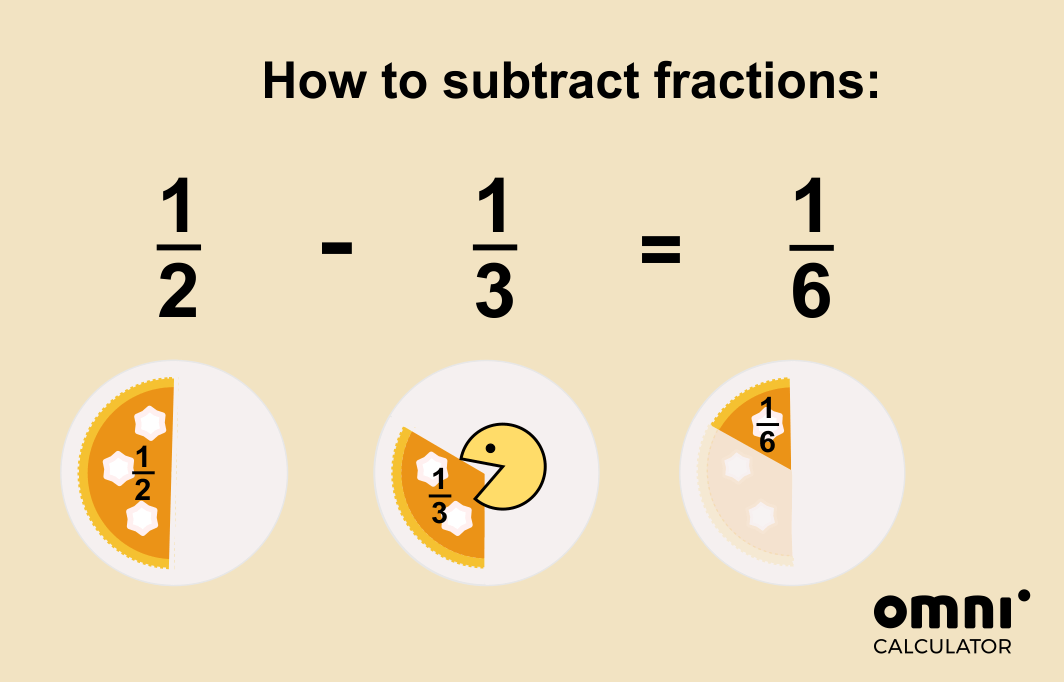That wasn't so hard, was it?

## How do you multiply fractions ✖️

Multiplying fractions is - fortunately - an effortless operation. It's numerator times numerator over denominator times denominator. Sometimes you also need to simplify the fraction. And that's it!

Take a look at this example:

2/3 * 5/6 = (2 * 5)/(3 * 6) = 10/18

which you can then simplify to 5/9

Whenever you deal with mixed fractions, don't forget than you must always write them as an improper number before multiplying:

2 1/2 * 3 1/4 = 5/2 * 13/4 = (5 * 13)/(2 * 4) = 65/8 =

8 1/8

And when multiplying a fraction by a whole number, remember that you can write the whole number as itself divided by 1:

3 * 5/7 = 3/1 * 5/7 = (3 * 5)/(1 * 7) = 15/7

If you don't know how to deal with fraction reduction, scroll down to the section .

Now that you know how do you multiply fractions, let's proceed to the next topic, fraction division.

## How to divide fractions ➗

Racking your brain over how to divide fractions? Have no worries! The division of fractions is quite similar to fraction multiplication. The only difference is that you must multiply your first number by the reciprocal of the second fraction. It may sound a bit weird, but it's really simple! Have a look at this example:

 (1/2) / (3/5) = 1/2 * 5/3 = (1 * 5)/(2 * 3) = 5/6

So, all you need to do is turn the second fraction upside down (which is its reciprocal) and multiply the fractions. Sometimes, you may also need to do some fraction reduction. And that's all, tadaaa!🎉

## How to simplify fractions?

We always like to make our lives simpler - even in maths. That's why simplifying fractions is such an important thing. It means that we write the fraction in its simplest possible form. We also call simplifying fractions reducing fractions.

But what does this mean, exactly? Have a look at these examples:

• You'd rather say that one-fourth (a quarter) of the pizza is left, instead of two-eights, right?
• or half of a cake was eaten, not three-sixths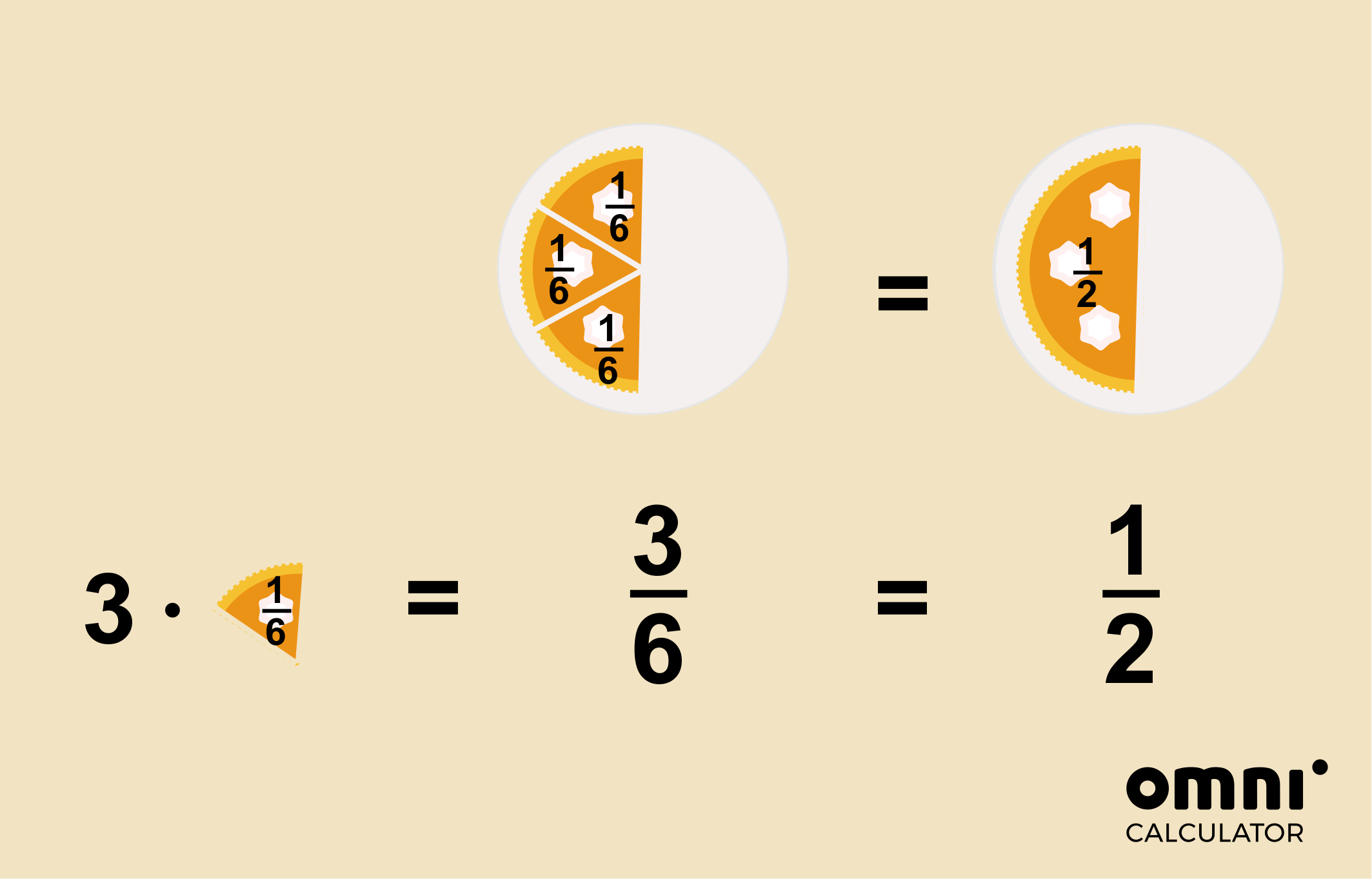To simplify a fraction, you can use two methods:

1. Use a kind of brute force approach: divide the fraction by 2,3,5,7,11... until it's impossible to continue the division (without a remainder):

42/126 = /:2

21/63 = /:3

7/21 = /:7

1/3

1. Find the GCF - greatest common factor of the numerator and denominator, and then divide them by this number:

GCF(42,126) = 42

42/126 = /:42

1/3

Read more about reducing fractions in our dedicated simplify fractions calculator. Also, make sure to have a look at our amazing equivalent fractions calculator, which can find many equivalents to your fraction.

## How to convert a decimal into fraction?

If you want to find out how to convert a decimal into a fraction, you're in the right place. Here's a step-by-step guide:

Let's assume you want to convert 0.32 to a fraction:

1. Treat your decimal as a numerator. Then, the denominator will be 1.

2. Move the decimal dot to the right, till you only have a whole number:

0.32 → 3.2 → 32

Each move corresponds to multiplication by 10

0.32 * 10 → 3.2 * 10 → 32

1. You multiplied the numerator by 10 * 10, and now we need to multiply the denominator by the same number:

1 * 10 * 10 = 100

1. You've changed your fraction to a decimal!✨ 0.32 → 32/100

2. Finally, simplify the result. As the greatest common factor for 32 and 100 is 4, divide both numerator and denominator by this value:

32/100 = 8/25

And that's it, the fraction in its simplest form. ❤️

But, if you have a repeating decimal... that's a different story. Have a good read of the section , or simply use our fraction calculator.

## How to turn a fraction into a decimal?

Well, the easiest way to turn a fraction into a decimal is... to use a calculator. Be it this fraction calculator, a standard pocket calculator🖩, or our dedicated tool - the fraction to decimal converter.

Sometimes the fraction is relatively easy to change into decimal without any tools - like for 1/2, 3/4 (or even 1/8). We believe that you can figure out how to expand the above fractions to have 10, 100, 1000, and so on in the denominator, respectively:

• Multiply 1/2 by 5, to get 10 as the denominator:

1/2 = 5/10 = 0.5

• Multiply 3/4 by 25, to get 100 as the denominator:

3/4 = 75/100 = 0.75

• Multiply 1/8 by 125, to get 1000 as the denominator:

1/8 = 125/1000 = 0.125

But what if you don't have the Internet or a calculator with you, but only pen and paper📝? And your fraction doesn't look so easy to expand as the ones above? Then, you'll probably need to do the long division to the decimal places by hand. Good luck! 🤞

Hanna Pamuła, PhD candidate
I want to
They are in
simple fraction form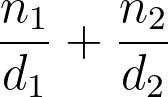1st fraction
Numerator (n₁)
Denominator (d₁)
2nd fraction
Numerator (n₂)
Denominator (d₂)
Result
If you want to add more than two fractions, or want a step by step solution, check our adding fractions calculator.
People also viewed…

### Characteristic polynomial

Characteristic polynomial calculator helps you determine the characteristic polynomial of any matrix of size 2×2, 3×3, or 4×4.

### Chilled drink

With the chilled drink calculator you can quickly check how long you need to keep your drink in the fridge or another cold place to have it at its optimal temperature. You can follow how the temperature changes with time with our interactive graph.

### Law of cosines

Use this law of cosines calculator to determine the third side of a triangle knowing two sides and the angle between them or to find the angles given all three sides.

### Sleep

The sleep calculator can help you determine when you should go to bed to wake up happy and refreshed.